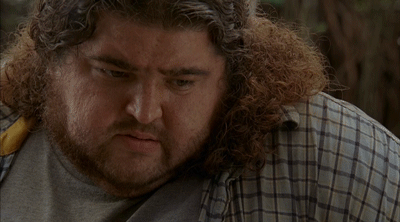My Reaction When The Multiplicative Inverse Property states that for every number a except 0, there is a number a^-1 = 1/a, such that a x = x a=1.
added 3 years ago